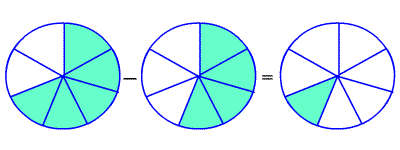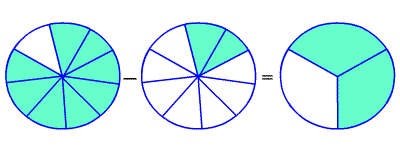# Subtracting Fractions with Like Denominators

Fractions with the same denominators are called like fractions.

To subtract fractions with like denominators, subtract the numerators , and write the difference over the denominator.

$\frac{5}{7}-\frac{4}{7}=\frac{1}{7}$Example :

Find $\frac{4}{5}-\frac{2}{5}$ .

Since the denominators are the same, subtract the numerators.

$=\frac{4\text{\hspace{0.17em}}-\text{\hspace{0.17em}}2}{5}$

$=\frac{2}{5}$

You may get an answer which is not in lowest terms , even if the fractions you were adding and subtracting both were. In this case, you have to reduce the fraction .

$\frac{8}{9}-\frac{2}{9}=\frac{6}{9}=\frac{6\text{\hspace{0.17em}}÷\text{\hspace{0.17em}}3}{9\text{\hspace{0.17em}}÷\text{\hspace{0.17em}}3}=\frac{2}{3}$